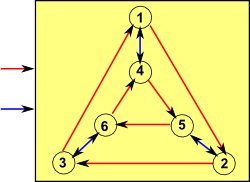# Finite State Machine / Finite State Automation

A finite state machine or automation provides a simple model of computation, a way to reduce the complexities of a computer to its essence, in addition to its theoretical significance this can be a good way to tackle certain types of practical problem.The current state is determined by past states of the system and the inputs to the system (it has a memory). A transition indicates a state change and is described by a condition that would need to be fulfilled to enable the transition. An action is a description of an activity that is to be performed at a given moment.

#### State diagram

We could describe the machine in terms of transitions, for each combination of state and input this will determine the transition function which gives the next state:#### State transition table

Condition↓Current state -> State 1 State 2 State 3 State 4 State 5 State 6
Input red State 2 State 3 State 1 State 5 State 6 State 4
Input blue State 4 State 5 State 6 State 1 State 2 State 3

### Outputs

There are different variants, for instance,

• Moore machine - machines having actions (outputs) associated with states.
• Mealy machine - machines having actions (outputs) associated with transitions.

### Mathematical Model

In its essence the model consists of (Σ,S,s0,δ), where:

• Σ is the input alphabet (a finite, non-empty set of symbols).
• S is a finite, non-empty set of states.
• s0 is an initial state, an element of S.
• δ is the state-transition function: δ: S × Σ -> S (in a nondeterministic finite state machine it would be δ: S × Σ -> (S1,S2,S3,S4) i.e., δ would return a set of states).

In some circumstances (for instance Mealy machine) we may need to add:

• F - the set of final states (subset of S) states, if any that result in the machine stopping.
• Γ - the output alphabet (a finite, non-empty set of symbols).
• ω - the output function - a mapping from the states to output.